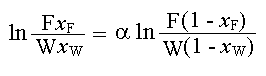### Differential Distillation

A binary mixture of benzene and toluene containing 40% of benzene is to be distilled at 1 atm pressure to recover 95% of the benzene. Estimate the molal percent of mixture to be distilled and the composition of the distillate obtained if distillation is carried out by Differential distillation, collecting all the distillate together.

The average relative volatility of benzene to toluene is 2.5.

Calculations:

Basis: 1 mol of feed

Formula:

For an ideal mixture of constant relative volatility, the following equation is used to find the number of moles present in the residue to the moles present initially in the feed:

nB/noB= (nA/noA)(1/αAB) (Reference: Example 18.6 in page no: 508 'Unit Operations of Chemical Engineering, McCabe-Smith, Fourth Edition')

Given:

noA = 0.4 mol

noB = 0.6 mol

nA = (1 - 0.95) x 0.4 = 0.02 mol

αAB = 2.5

Substituting,

nB = 0.6 x (0.02/0.4)(1/2.5) = 0.181 mol

Composition of residue:

xW = 0.02/(0.02 + 0.181) = 0.0995

Composition of distillate:

Moles of benzene in distillate = moles of benzene in feed - moles of benzene in residue

= 0.4 - 0.02 = 0.38

Moles of toluene in distillate = 0.6 - 0.181 = 0.419

xD = 0.38/(0.38 + 0.419) = 0.4756

Mole percent of mixture to be distilled = 100 x (0.38 + 0.419)/1 = 79.9%

The above calculations can also done with the modification of Rayleigh's equation for constant relative volatility system:Basis: 1 mole of feed mixture

Given:

Moles of Benzene present in the feed = 0.4 = FxF

95% of benzene that was present in the feed is to be recovered in the distillate. Therefore, moles of Benzene present in the residue = (1 - 0.95) x 0.4 = 0.05 x 0.4 = 0.02 = WxW

And xF = 0.4; α = 2.5

F(1 - xF) = F - FxF = 1 - 0.4 = 0.6

Substituting the known quantities in the above equation,

ln (0.4/0.02) = 2.5 x ln [0.6/(W (1 - xW))]

(0.4/0.02)1/2.5 = 0.6 / [W(1 - xW)]

3.31445 = 0.6 / [W(1 - xW)]

W(1 - xW) = 0.181

Therfore, W = W(1 - xW) + WxW = 0.181 + 0.02 = 0.201

Moles percent of mixture to be distilled = F - W = 1 - 0.201 = 0.799

Mole percent of mixture to be distilled = 0.799 x 100 = 79.9%

Composition of distillate:

Moles of toluene in residue = W(1 - xW) = 0.181

Moles of toluene in distillate = moles of toluene in feed - mole of toluene in residue = 0.6 - 0.181 = 0.419

Moles of benzene in distillate = moles of benzene in feed - moles of benzene in residue = 0.4 - 0.02 = 0.38

Mole fraction of benzene in distillate = 0.38 / (0.38 + 0.419) = 0.4756